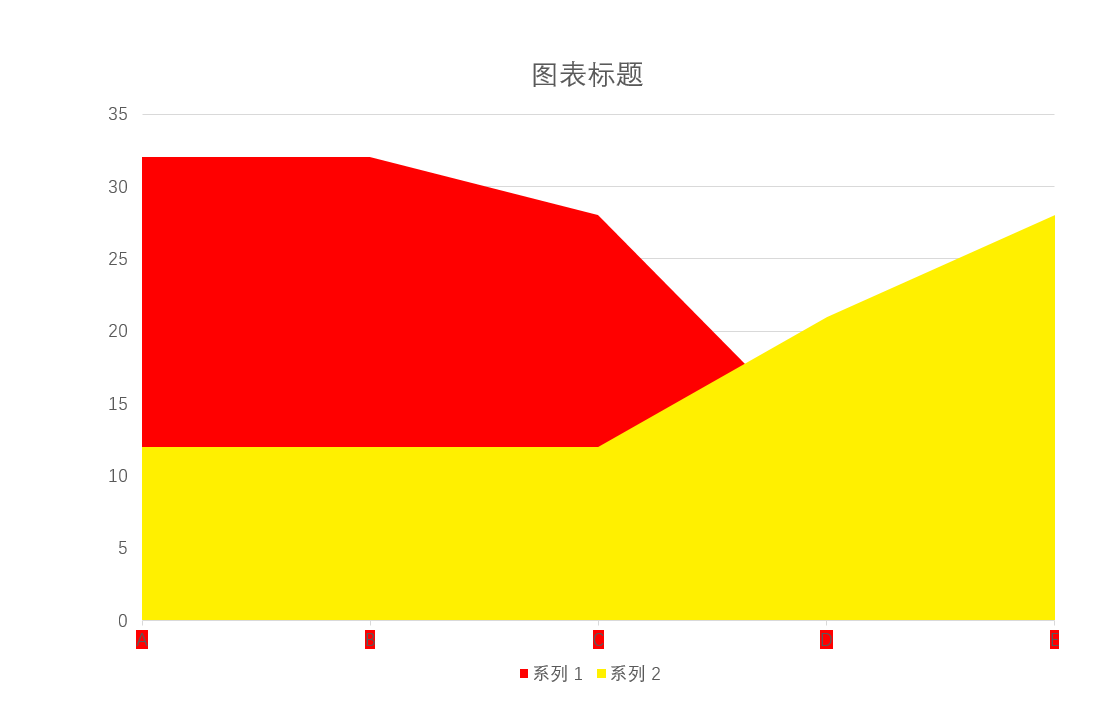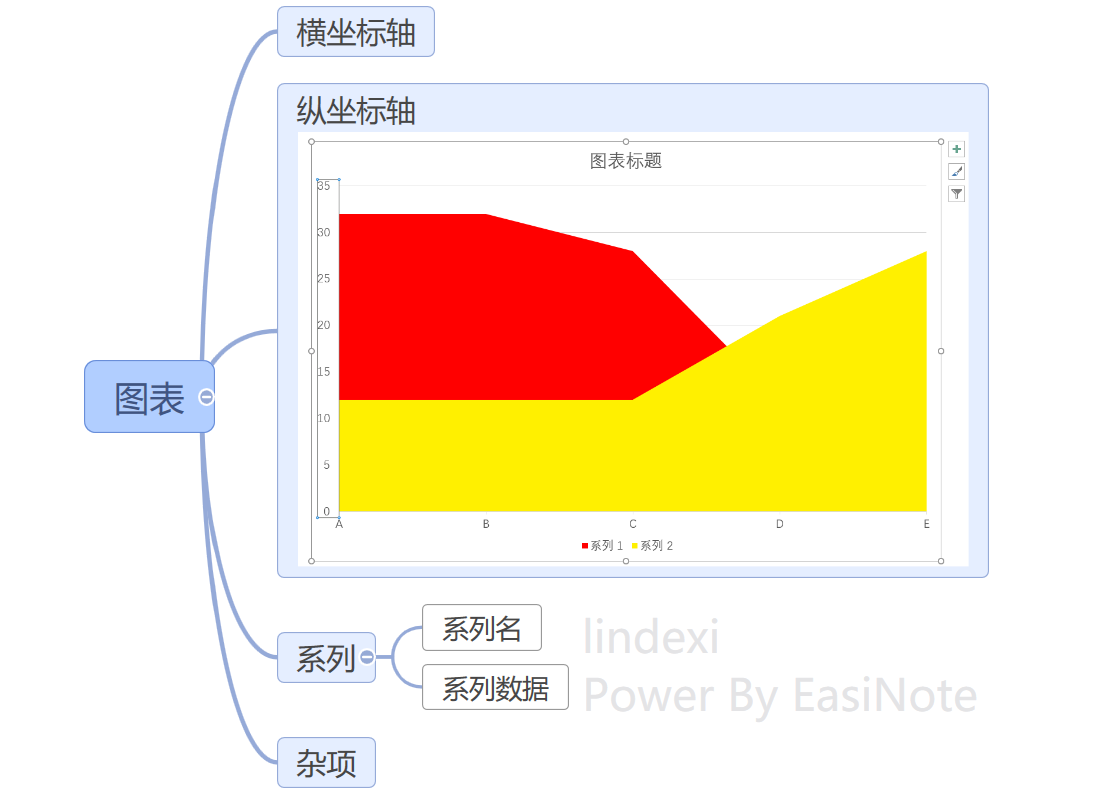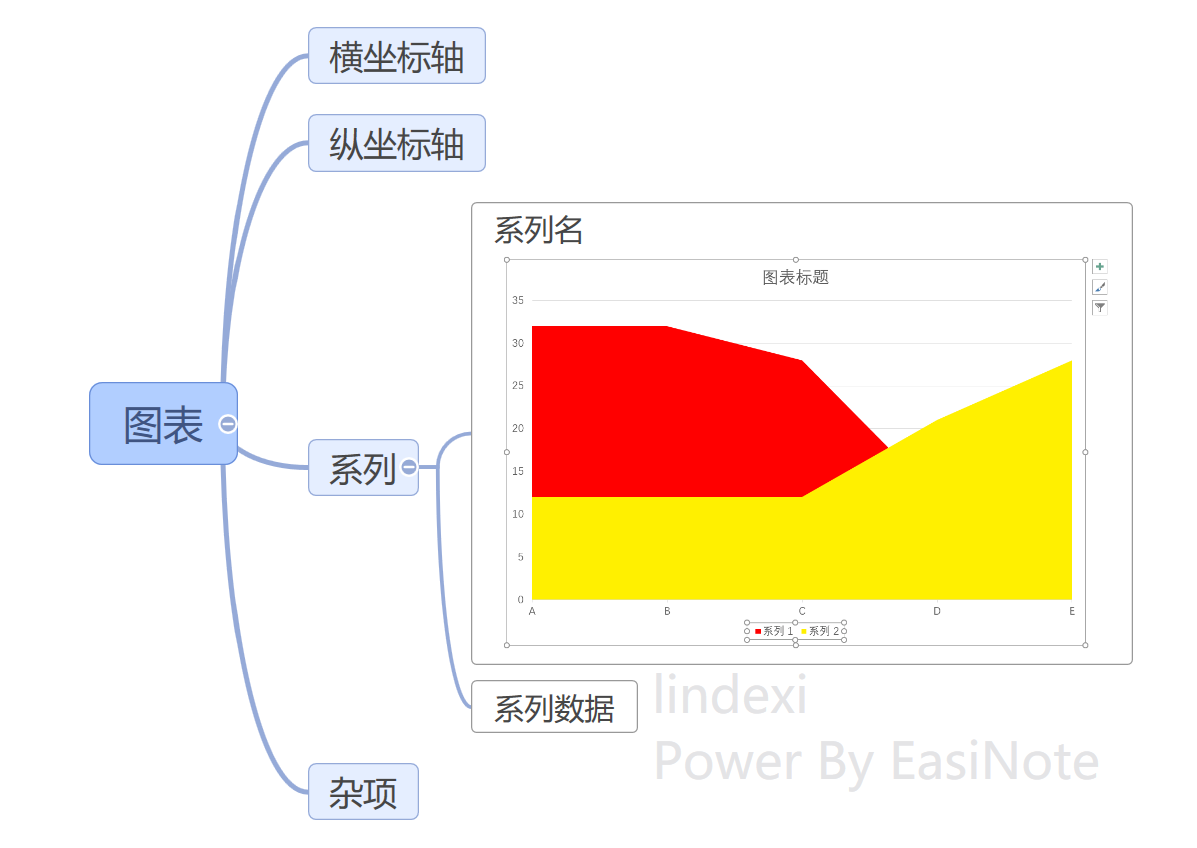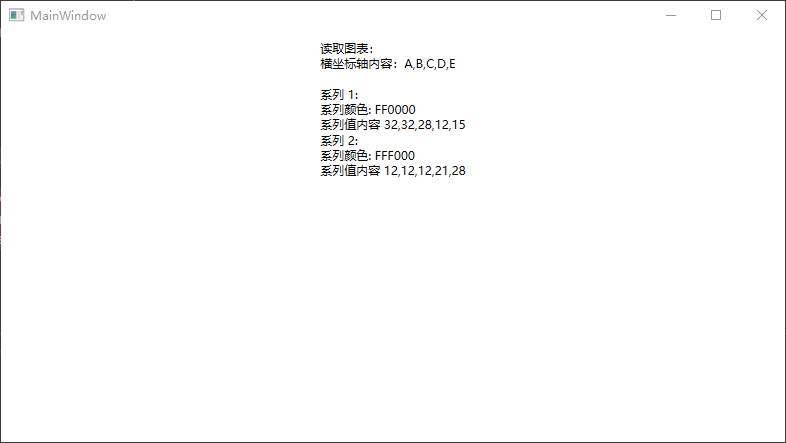﻿ dotnet OpenXML 解析 PPT 圖表 面積圖入門_ZenDei技術網路在線

# dotnet OpenXML 解析 PPT 圖表 面積圖入門## 圖表的組成

### 橫坐標軸 類別坐標軸數據### 縱坐標軸### 數據系列``````系列 1：32,32,28,12,15

``````

## 效果## 讀取圖表

``````            var file = new FileInfo("Test.pptx");

using var presentationDocument = PresentationDocument.Open(file.FullName, false);
``````

``````            var slide = presentationDocument.PresentationPart!.SlideParts.First().Slide;
``````

`````` <p:cSld>
<p:spTree>
<p:graphicFrame>
...
</p:graphicFrame>
</p:spTree>
</p:cSld>
``````

``````            // 獲取圖表元素，在這份課件里，有一個面積圖。以下使用 First 忽略細節，獲取圖表
var graphicFrame = slide.Descendants<GraphicFrame>().First();
// 獲取到對應的圖表信息，圖表是引用的，內容不是放在 Slide 頁面裡面，而是放在獨立的圖表 xml 文件里
var graphic = graphicFrame.Graphic;
var graphicData = graphic?.GraphicData;
var chartReference = graphicData?.GetFirstChild<DocumentFormat.OpenXml.Drawing.Charts.ChartReference>();
``````

``````   <p:graphicFrame>
<a:graphic>
<a:graphicData uri="http://schemas.openxmlformats.org/drawingml/2006/chart">
<c:chart xmlns:c="http://schemas.openxmlformats.org/drawingml/2006/chart" xmlns:r="http://schemas.openxmlformats.org/officeDocument/2006/relationships" r:id="rId2" />
</a:graphicData>
</a:graphic>
</p:graphicFrame>
``````

``````            // 獲取到 id 也就是 `r:id="rId2"` 根據 Relationship 的描述，可以知道去 rels 文件裡面獲取關聯的內容。在 OpenXml SDK 里，封裝好了獲取方法，獲取時需要有兩個參數，一個是 id 另一個是去哪裡獲取的 Part 內容
var id = chartReference?.Id?.Value;
``````

``````            // 如果是放在模版裡面，記得要用模版的 Part 去獲取
var currentPart = slide.SlidePart!;

if (!currentPart.TryGetPartById(id!, out var openXmlPart))
{
// 在這份課件里，一定不會進入此分支
// 一定能從頁面找到對應的資源內容也就是圖表
return;
}
``````

``````            if (openXmlPart is not ChartPart chartPart)
{
// 這裡拿到的一定是 ChartPart 對象，一定不會進入此分支。但是在實際項目的代碼，還是要做這個判斷
return;
}
``````

`````` <?xml version="1.0" encoding="UTF-8" standalone="yes"?>
<c:chartSpace>
<c:chart>
...
<c:plotArea>
...
</c:plotArea>
</c:chart>
</c:chartSpace>
``````

``````using Chart = DocumentFormat.OpenXml.Drawing.Charts.Chart;

// 這裡的 ChartPart 對應的就是 charts\chartN.xml 文件。這裡的 chartN.xml 表示的是 chart1.xml 或 chart2.xml 等文件
var chartSpace = chartPart.ChartSpace;

// 這裡的 Chart 是 DocumentFormat.OpenXml.Drawing.Charts.Chart 類型，在 OpenXmlSDK 裡面，有多個同名的 Chart 類型，還請看具體的命名空間
/*
<?xml version="1.0" encoding="UTF-8" standalone="yes"?>
<c:chartSpace>
<c:chart>
...
<c:plotArea>
...
</c:plotArea>
</c:chart>
</c:chartSpace>
*/
var chart = chartSpace.GetFirstChild<Chart>();
``````

``````using PlotArea = DocumentFormat.OpenXml.Drawing.Charts.PlotArea;

var chart = chartSpace.GetFirstChild<Chart>();
var plotArea = chart?.GetFirstChild<PlotArea>();
``````

`````` <c:plotArea>
<c:areaChart>
...
</c:areaChart>
</c:plotArea>
``````

``````            var areaChart = plotArea?.GetFirstChild<AreaChart>();

if (areaChart == null)
{
// 在這份課件里，一定存在面積圖，一定不會進入此分支
return;
}
``````

``````  <c:plotArea>
<c:areaChart>
<c:ser>
...
</c:ser>
<c:ser>
...
</c:ser>
</c:areaChart>
</c:plotArea>
``````

• 系列名
• 系列數據
• 類別軸上的數據
• 樣式信息

``````            foreach (var areaChartChildElement in areaChart.ChildElements)
{
// 獲取系列
/*
<c:plotArea>
<c:areaChart>
<c:ser>
...
</c:ser>
<c:ser>
...
</c:ser>
</c:areaChart>
</c:plotArea>
*/
if (areaChartChildElement is DocumentFormat.OpenXml.Drawing.Charts.AreaChartSeries areaChartSeries)
{
// 類別軸上的數據 橫坐標軸上的數據
var categoryAxisData = areaChartSeries.GetFirstChild<DocumentFormat.OpenXml.Drawing.Charts.CategoryAxisData>()!;
}
}
``````

`````` <c:plotArea>
<c:areaChart>
<c:ser>
<c:cat>
</c:cat>
</c:ser>
</c:areaChart>
</c:plotArea>
``````

``````var categoryAxisData = areaChartSeries.GetFirstChild<DocumentFormat.OpenXml.Drawing.Charts.CategoryAxisData>()!;
var categoryAxisDataStringReference = categoryAxisData.GetFirstChild<StringReference>();
``````

``````  <c:cat>
<c:strRef>
<c:f>Sheet1!\$A\$2:\$A\$6</c:f>
<c:strCache>
<c:ptCount val="5" />
<c:pt idx="0">
<c:v>A</c:v>
</c:pt>
<c:pt idx="1">
<c:v>B</c:v>
</c:pt>
<c:pt idx="2">
<c:v>C</c:v>
</c:pt>
<c:pt idx="3">
<c:v>D</c:v>
</c:pt>
<c:pt idx="4">
<c:v>E</c:v>
</c:pt>
</c:strCache>
</c:strRef>
</c:cat>
``````

`````` var categoryAxisDataStringReference = categoryAxisData.GetFirstChild<StringReference>();
if (categoryAxisDataStringReference != null)
{
// 這個公式表示是從 Excel 哪個數據獲取的，獲取的方式比較複雜。這裡還是先從緩存獲取
var categoryAxisDataFormula = categoryAxisDataStringReference.GetFirstChild<DocumentFormat.OpenXml.Drawing.Charts.Formula>();
}
``````

``````  // 讀取緩存
var categoryAxisDataStringCache = categoryAxisDataStringReference.GetFirstChild<DocumentFormat.OpenXml.Drawing.Charts.StringCache>()!;
``````

`````` var list = new List<string>();
foreach (var stringPoint in categoryAxisDataStringCache.Elements<DocumentFormat.OpenXml.Drawing.Charts.StringPoint>())
{
// 以下的 類別軸上的數據 橫坐標軸上的數據，各個列項的名稱
// 對於面積圖來說，多個系列的列項都是相同的。儘管在 OpenXml 存儲裡面存放了兩份，但以第零個系列的為準
var text = stringPoint.GetFirstChild<DocumentFormat.OpenXml.Drawing.Charts.NumericValue>()!.Text;

}
``````

``````  // 獲取系列標題，放心，可以不讀取 Excel 的內容，通過緩存內容即可。但是緩存內容也許和 Excel 內容不對應
/*
<c:plotArea>
<c:areaChart>
<c:ser>
<c:tx>
<c:strRef>
<c:f>Sheet1!\$B\$1</c:f>
<c:strCache>
<c:ptCount val="1" />
<c:pt idx="0">
<c:v>系列 1</c:v>
</c:pt>
</c:strCache>
</c:strRef>
</c:tx>
...
</c:ser>
</c:areaChart>
</c:plotArea>
*/
var seriesText = areaChartSeries.GetFirstChild<DocumentFormat.OpenXml.Drawing.Charts.SeriesText>()!;
var seriesTextStringReference = seriesText.GetFirstChild<DocumentFormat.OpenXml.Drawing.Charts.StringReference>()!;
// 這個公式表示是從 Excel 哪個數據獲取的，獲取的方式比較複雜。這裡還是先從緩存獲取
var seriesTextFormula = seriesTextStringReference.GetFirstChild<DocumentFormat.OpenXml.Drawing.Charts.Formula>();
``````

`````` // 有緩存的話，從緩存獲取就可以，緩存內容也許和 Excel 內容不對應
/*
<c:strCache>
<c:ptCount val="1" />
<c:pt idx="0">
<c:v>系列 1</c:v>
</c:pt>
</c:strCache>
*/
var seriesTextStringCache = seriesTextStringReference.GetFirstChild<DocumentFormat.OpenXml.Drawing.Charts.StringCache>();
if (seriesTextStringCache != null)
{
var seriesTextStringPoint = seriesTextStringCache.GetFirstChild<DocumentFormat.OpenXml.Drawing.Charts.StringPoint>();

var numericValue = seriesTextStringPoint!.GetFirstChild<DocumentFormat.OpenXml.Drawing.Charts.NumericValue>();
// 系列1 標題
var title = numericValue!.Text;

}
``````

``````  <c:plotArea>
<c:areaChart>
<c:ser>
<c:tx>
...
</c:tx>
<c:spPr>
<a:solidFill>
<a:srgbClr val="FF0000" />
</a:solidFill>
</c:spPr>
</c:ser>
</c:areaChart>
</c:plotArea>
``````

``````  // 圖表的形狀屬性的內容和 形狀屬性 的內容是差不多的
/*
<c:plotArea>
<c:areaChart>
<c:ser>
<c:tx>
...
</c:tx>
<c:spPr>
<a:solidFill>
<a:srgbClr val="FF0000" />
</a:solidFill>
</c:spPr>
</c:ser>
</c:areaChart>
</c:plotArea>
*/
var chartShapeProperties = areaChartSeries.GetFirstChild<DocumentFormat.OpenXml.Drawing.Charts.ChartShapeProperties>()!;
// 獲取畫刷，畫刷有好多不同的類型，這個課件只用了純色
var solidFill = chartShapeProperties.GetFirstChild<SolidFill>()!;
// 畫刷純色顏色有很多個顏色表示方法，這個課件只用了 RGB 的純色
var rgbColorModelHex = solidFill.GetFirstChild<DocumentFormat.OpenXml.Drawing.RgbColorModelHex>()!;
// 這就是這個系列的顏色
var colorValue = rgbColorModelHex.Val!.Value;
``````

`````` // 獲取系列的值
/*
<c:plotArea>
<c:areaChart>
<c:ser>
<c:tx>
...
</c:tx>
<c:cat>
...
</c:cat>
<c:val>
<c:numRef>
<c:f>Sheet1!\$B\$2:\$B\$6</c:f>
<c:numCache>
<c:formatCode>General</c:formatCode>
<c:ptCount val="5" />
<c:pt idx="0">
<c:v>32</c:v>
</c:pt>
<c:pt idx="1">
<c:v>32</c:v>
</c:pt>
<c:pt idx="2">
<c:v>28</c:v>
</c:pt>
<c:pt idx="3">
<c:v>12</c:v>
</c:pt>
<c:pt idx="4">
<c:v>15</c:v>
</c:pt>
</c:numCache>
</c:numRef>
</c:val>
</c:ser>
<c:ser>
...
</c:ser>
</c:areaChart>
</c:plotArea>
*/
// 這就是系列裡面最重要的數據。然而在 PPT 裡面，是允許為空的，如果是空，行為就是不繪製系列內容
var valueList = new List<string>();
var values = areaChartSeries.GetFirstChild<DocumentFormat.OpenXml.Drawing.Charts.Values>();
``````

``````  var valuesNumberReference = values?.GetFirstChild<DocumentFormat.OpenXml.Drawing.Charts.NumberReference>();
if (valuesNumberReference != null)
{
/*
<c:val>
<c:numRef>
<c:f>Sheet1!\$B\$2:\$B\$6</c:f>
<c:numCache>
<c:formatCode>General</c:formatCode>
<c:ptCount val="5" />
<c:pt idx="0">
<c:v>32</c:v>
</c:pt>
<c:pt idx="1">
<c:v>32</c:v>
</c:pt>
<c:pt idx="2">
<c:v>28</c:v>
</c:pt>
<c:pt idx="3">
<c:v>12</c:v>
</c:pt>
<c:pt idx="4">
<c:v>15</c:v>
</c:pt>
</c:numCache>
</c:numRef>
</c:val>
*/
// 這份課件一定存在 values 內容
// 和其他的一樣，存在引用 Excel 的內容。這裡同樣也是採用緩存
var valuesFormula = valuesNumberReference.GetFirstChild<DocumentFormat.OpenXml.Drawing.Charts.Formula>();
``````

``````  var valuesNumberingCache = valuesNumberReference.GetFirstChild<DocumentFormat.OpenXml.Drawing.Charts.NumberingCache>()!;

// 通過 FormatCode 決定界面效果。這份課件是 General 表示不用格式化
var formatCode = valuesNumberingCache.FormatCode;
Debug.Assert(formatCode?.Text == "General");
``````

`````` var valueList = new List<string>();
foreach (var numericPoint in valuesNumberingCache.Elements<DocumentFormat.OpenXml.Drawing.Charts.NumericPoint>())
{
var numericValue = numericPoint.GetFirstChild<DocumentFormat.OpenXml.Drawing.Charts.NumericValue>()!;
var numericValueText = numericValue.Text;

}
``````

## 數據存儲

``````    <c:plotArea>
<c:areaChart>
<c:ser>
<!-- 系列的數據 -->
</c:ser>
<c:ser>
<c:tx>
<!-- 系列標題 -->
</c:tx>
<c:spPr>
<!-- 系列樣式 -->
</c:spPr>
<c:cat>
<!-- 類別軸上的數據 -->
</c:cat>
<c:val>
<!-- 系列數據 -->
</c:val>
</c:ser>
</c:areaChart>
</c:plotArea>
``````

## 代碼

``````git init
git pull origin 2f266d20916f784662d84a98d60b7e1bd097d11d
``````

``````git remote remove origin
``````

## 更多• 簡介 FTP是FileTransferProtocol（文件傳輸協議）的英文簡稱，而中文簡稱為“文傳協議”。用於Internet上的控制文件的雙向傳輸。同時，它也是一個應用程式（Application）。基於不同的操作系統有不同的FTP應用程式，而所有這些應用程式都遵守同一種協議以傳輸文件。 FTP ...
• 在繼承中，派生類可以拿到基類的方法，若是派生類很多，且有時某部分派生類的部分實現邏輯是一樣的，但其他的派生類又用不到，這個時候這些邏輯若是全部寫到派生類中，就會導致產生很多的重覆邏輯，但是若是寫到基類中就會導致其他用不到當前邏輯的派生類也能調用，這樣就會導致代碼維護出現了問題。由此產生了介面。 在C ...
• WPF 截圖控制項之移除控制項(九)「仿微信」 WPF 截圖控制項之移除控制項(九)「仿微信」 作者：WPFDevelopersOrg 原文鏈接： https://github.com/WPFDevelopersOrg/WPFDevelopers 框架使用大於等於.NET40； Visual Studio ...
• 從零開始搭建基於 ABP Framework 分層架構解決方案，快速集成框架內置應用模塊。探索基於 ABP Framework 極速開發的最佳路徑，構建一個模塊完備、可開發、可調試、可發佈和部署的分層架構解決方案。 ...
• 一、前言 之前分享過一期關於DrawingVisual來繪製高性能曲線的博客，今天再分享一篇通過另一種方式來繪製高性能曲線的方法，也就是通過WriteableBitmap的方式；具體的一些細節這裡就不啰嗦了，同樣是局部繪製的思想，滾動條拖動到哪裡，就只繪製那一部分的曲線，直接貼代碼；（該程式在英特爾 ...
• 規則，點擊投籃目標點，就會有一個球沿著相關拋物線，然後，判斷是否進入籃子里，其實就是一個矩形，直接是按照碰撞檢測來的，碰到就算進去了，對其增加了一個分數統計等功能。 ...